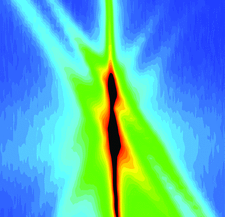# issue contentsFOUNDATIONSADVANCES
ISSN: 2053-2733

# May 2004 issueCover illustration: Diffuse scattering about the 002 reflection of an AlAs/GaAs superlattice grown on an oriented GaAs substrate. The in-plane and out-of plane components of the momentum transfer vector are oriented horizontally and vertically, respectively. The observed features are explained on the basis of the distorted-wave Born approximation. See Chernov et al. [Acta Cryst. (2003), A59, 551-559].

## research papers

### A strict solution for the optimal superimposition of protein structures

Existing methods for the optimal superimposition of two protein molecules are based on the precondition that the centroids of the vector sets of their atomic coordinates are coincident. This is obviously not strict in the mathematical sense. The theorem presented in this paper is a strict solution for this problem in the mathematical sense.

### A comparison between experimental and theoretical aspherical-atom scattering factors for charge-density refinement of large molecules

Two databases of multipoles describing the electron density of the polypeptide main chain are compared.

### X-ray multiple-wave coherent interaction in a quasi-two-dimensional material NbSe2-2H

The first observation of X-ray multiple-wave interaction in an incommensurate charge-density-wave (CDW) modulated structure at low temperatures is reported for an example of a quasi-two-dimensional material, NbSe2-2H.

### On the equivalence between a commonly used correlation coefficient and a least-squares function

It is established that the set of global maximizers of a commonly used correlation coefficient are also global minimizers of a least-squares function.

### Twinning by reticular pseudo-merohedry in trigonal, tetragonal and hexagonal crystals

Twin laws by (reticular) pseudo-merohedry are investigated for which the obliquity δ vanishes for an appropriate value of the axial ratio c/a. For arbitrary values of c/a, it is shown that δ is related to the deformation parameter ∊ introduced by Bonnet & Durand [Philos. Mag. (1975>), 32, 997–1006]. Furthermore, tanδ is a product of two factors: one describes the dependency on the lattice parameters, the other the effect of the lattice misorientation.

### Locating heavy atoms by integrating direct methods and SIR techniques

The joint probability distribution method has been applied to locate heavy atoms in the isomorphous replacement method.

### Exceptional properties of some sphere packings in the general position of P6222

Three types of sphere packing with remarkable properties are described. They show that from the crystallographic point of view in exceptional cases the usual definition of sphere-packing types based on graph-theoretical criteria may be not sufficient.

### Minimal densities of cubic sphere-packing types

Minimal sphere-packing densities and sample coordinates are presented for all 199 types of homogeneous sphere packings with cubic symmetry.

### Atomic displacement parameters and anisotropic thermal ellipsoid lengths and angles

The moments of the probability distribution of n-dimensional anisotropic thermal ellipsoids are provided as functions of dimension n. For specific dimensions n = 2 and n = 3, new formulas are presented for the semi-axis lengths and rotation angles of the thermal ellipsoids.

### Crystal structures and cellular automata

Cellular automata are used to model the topology of structures consisting of frameworks based upon fundamental building blocks.

### The structure of the cubic coincident site lattice rotation group

The mathematical relationships among rotations producing coincident site lattices are developed in terms of intuitively understandable algebraic and topological models. Examples demonstrate applications in grain-boundary engineering and in the statistical descriptions of grain-boundary networks.

## short communications

### A theorem on the fullerenes with no adjacent pentagons

The theorem that a fullerene Cn with any even n ≥ 70 and no adjacent pentagons exists is proved.

### Polarization factors in the symmetrical case of three-wave diffraction

The symmetrical case of multiple diffraction, where a triplet of the diffraction vectors forms an isosceles triangle, is considered. A simple trigonometric form is obtained for the polarization factor by treating separately the variables for spectral-dependent and spectral-independent contributions.